# Starting Out with Time SeriesTime series analysis and forecasting find wide usage in the financial markets across assets like stocks, F&O, Forex, and Commodities. As such, it becomes pertinent for aspiring quants to have sound knowledge in time series forecasting. In this post, we will introduce the basic concepts of time series and illustrate how to create time series plots and analysis in R programming language.

### Time series defined

A time series is a sequence of observations over time, which are usually spaced at regular intervals of time. For example:
• Daily stock prices for the last 5 years
• 1-minute stock price data for the last 90 days
• Quarterly revenues of a company over the last 10 years
• Monthly car sales of an automaker for the last 3 years
• Annual unemployment rate of a state in the last 50 years

### Univariate time series and Multivariate time series

A univariate time series refers to the set of observations over time of a single variable. Correspondingly, a multivariate time series refers to the set of observations over time of several variables.

### Time Series Analysis and Forecasting

In time series analysis, the objective is to apply/develop models which are able to describe the given time series with a fair amount of accuracy. On the other hand, time series forecasting involves forecasting the future values of a given time series using the past observed values. There are various models that are used for forecasting and the viability of a particular model used for forecasting is determined by its performance at predicting the future values.

Some examples of time series forecasting:

• Forecasting the closing price of a stock every day
• Forecasting the quarterly revenues of a company
• Forecasting the monthly number of cars sold.

### Plotting a time series

A plot of a time series data gives a clear picture of the spread over the given time period. It becomes easy for a human eye to detect any seasonality or abnormality in a given time series.

### Plotting a time series in R

To plot a time series in R, we first need to read the data in R. If the data is available in a CSV file or in an Excel file, we can read the data in R using the csv.read() function or the read.xlsx() function respectively. Once the data has been read, we can create a time series plot by using the plot.ts() function. See the example given below.

We will use the time series data set from the Time Series Data Library (TSDL) created by Rob Hyndman. We will plot the monthly closings of the Dow-Jones industrial index, Aug. 1968 – Oct. 1992. Save the dataset in your current R working directory with the name monthly-closings-of-the-dowjones.csv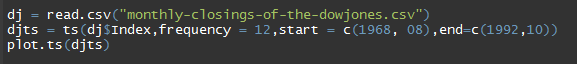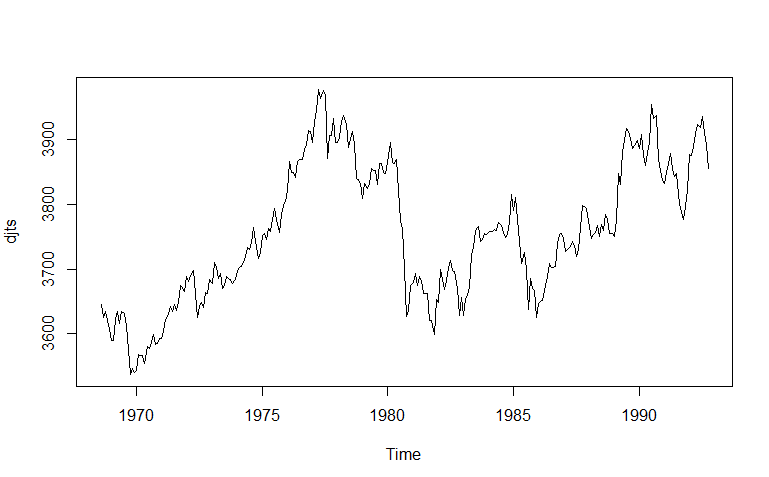### Decomposing time series

A time series generally comprises of a trend component, irregular (noise) component, and can also have a seasonal component, in the case of a seasonal time series. Decomposing time series means separating the original time series into these components.

Trend – The increasing or decreasing values in a given time series.

Seasonal – The repeating cycle over a specific period (day, week, month, etc.) in a given time series.

Irregular (Noise) – The random (irregularity) of values in a given time series

### Why do we need to decompose a time series?

As mentioned in the above paragraph, a time series might include a seasonal component or an irregular component. In such a case, we would not get a true picture of the trending property of the time series. Hence, we need to separate out the seasonality effect and/or the noise which will give us a clear picture, and help in further analysis.

### How do we decompose a time series?

There are two structures which can be used for decomposing a given time series.
1. Additive decomposition – If the seasonal variation is relatively constant over time, we can use the additive structure for decomposing a given time series. The additive structure is given as -

Xt = Trend + Random + Seasonal

1. Multiplicative decomposition – If the seasonal variation is increasing over time, we can use the multiplicative structure for decomposing a time series. The multiplicative structure is given as -

Xt = Trend * Random * Seasonal

### Decomposing a time series in R

To decompose a non-seasonal time series in R, we can use a smoothing method for calculating the moving average of a given time series. We can use the SMA() function from the TTR package to smooth out the time series.

To decompose a seasonal time series in R, we can use the decompose() function. This function estimates the trend, seasonal, and irregular (noise) components of a given time series. The decompose function is given as -

decompose(x, type = c("additive", "multiplicative"), filter = NULL)

Arguments
x - A time series
type - The type of seasonal component. Can be abbreviated
filter - A vector of filter coefficients in reverse time order (as for AR or MA coefficients), used for filtering out the seasonal component. If NULL, a moving average with the symmetric window is performed.

When we use the decompose function, we need to specify the trend type (multiplicative, additive) and seasonality type (multiplicative, additive) in the arguments.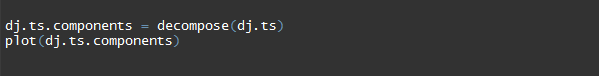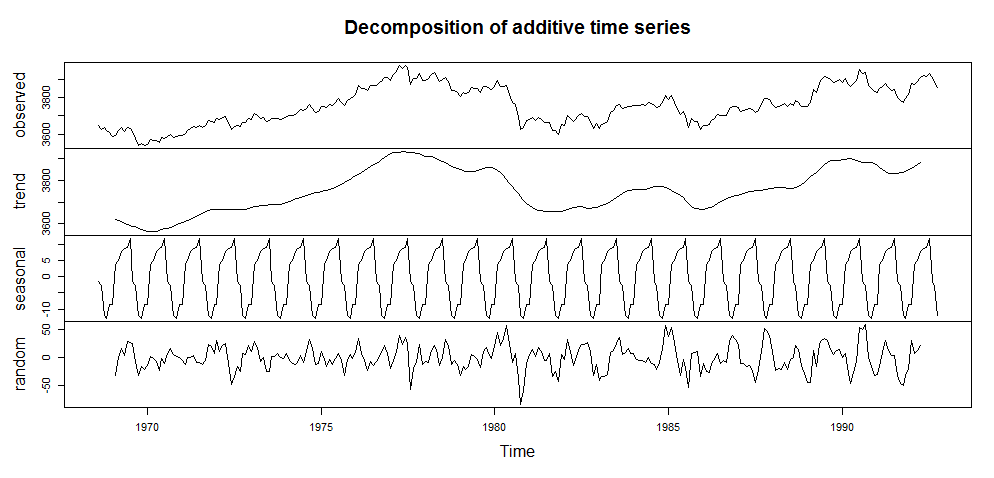### Stationary and non-stationary time series

A stationary time series is one where the mean and the variance are both constant over time or is one whose properties do not depend on the time at which the series is observed. Thus, the time series is a flat series without trend, constant variance over time, a constant mean, a constant autocorrelation and no seasonality. This makes a stationary time series is easy to predict. On the other hand, a non-stationary time series is one where either mean or variance or both are not constant over time.

There are different tests that can use to check whether a given time series is stationary. These include the Autocorrelation function (ACF), Partial autocorrelation function (PACF), Ljung-Box test, Augmented Dickey–Fuller (ADF) t-statistic test, and the Kwiatkowski-Phillips-Schmidt-Shin (KPSS) test.

Let us test our sample time series with the Autocorrelation function (ACF), Partial autocorrelation function (PACF) to check if it is stationary.

Autocorrelation function (ACF) – The autocorrelation function checks for correlation between two different data points of a time series separated by a lag “h”. For example, the ACF will check for correlation between points #1 and #2, #2 and #3 etc. Similarly, for lag 3, the ACF function will check between points #1 and #4, #2 and #5, #3 and #6 etc.

R code for ACF -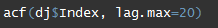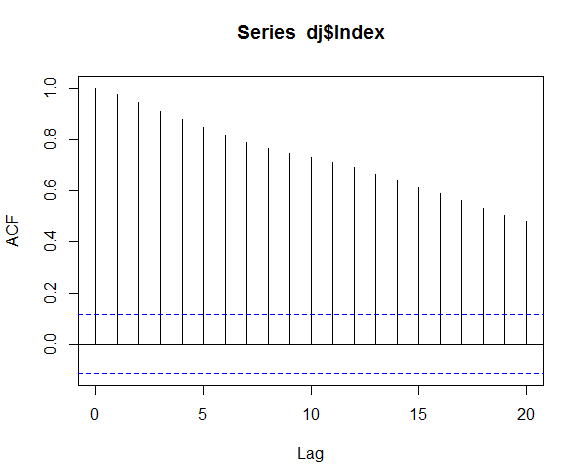Partial autocorrelation function (PACF) – In some cases, the effect of autocorrelation at smaller lags will have an influence on the estimate of autocorrelation at longer lags. For example, a strong lag one autocorrelation can cause an autocorrelation with lag three. The Partial Autocorrelation Function (PACF) removes the effect of shorter lag autocorrelation from the correlation estimate at longer lags.

R code for PACF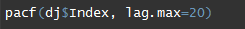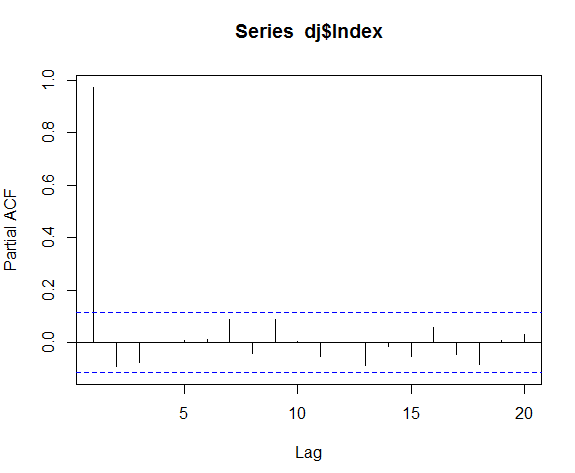The values of ACF and PACF each vary between plus and minus one. When the values are closer to plus or minus one it indicates a strong correlation. If the time series is stationary, the ACF will drop to zero relatively quickly, while the ACF of non-stationary time series will decrease slowly. From the ACF graph, we can conclude that the given time series in non-stationary.

### Conclusion

In this post, we gave an overview of time series, plotting time series data, and decomposition of a time series into its constituent components using R programming language. We also got introduced to the concept of stationary and non-stationary time series and the tests which can be carried out to check if the given time series is stationary. In our upcoming post, we will continue with the concept stationary time series and see how to convert a non-stationary time series into a stationary time series. For further reference, you might like go through the following: http://a-little-book-of-r-for-time-series.readthedocs.io/en/latest/src/timeseries.html

### Next Step

We have discussed some important tools that are really helpful for traders to analyse time series data and in designing and backtesting trading strategies. This blog focuses on how to deal with dates and frequency of the time series and performing Time Series Analysis by extensively using the ‘datetime’ library in Python.